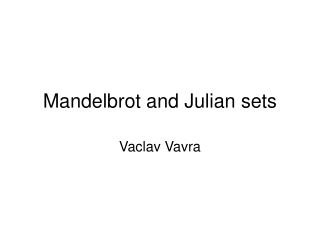DownloadDownload PresentationMandelbrot and Julian sets

# Mandelbrot and Julian sets

Download Presentation## Mandelbrot and Julian sets

- - - - - - - - - - - - - - - - - - - - - - - - - - - E N D - - - - - - - - - - - - - - - - - - - - - - - - - - -
##### Presentation Transcript

1. Mandelbrot and Julian sets Vaclav Vavra

2. Definitions • By both sets we compute the same sequence given by recursive formula: zn = (zn-1)2 + c • zn , zn-1, c are complex numbers(!) • Julian set is set of z0’s for which the sequence does not diverge • (c is constant for a given Julian set) • formally: J = C - {z0| zn = (zn-1)2 + c→ ∞} or J = {z0| ┐(zn = (zn-1)2 + c→ ∞)}

3. Definitions • Mandelbrot set is set of c’sfor which the sequence does not diverge for z0 = 0 • formally: J = C - {c|z0 = 0, zn = (zn-1)2 + c→ ∞} or J = {c|z0 = 0, ┐(zn = (zn-1)2 + c→ ∞)}

4. Operations in C • Notation: x = a + b.i • a is real part, b is imaginary part, i is imaginary unit, i2=-1 • Operations: y = c + d.i x + y = (a+c) + (b+d).i x.y = (ac−bd) + (bc+ad).i |x| = sqrt(a2 + b2)

5. Visualisation • for a given c, z0 we compute z1, z2, z3,…in a loop • this way we cannot analytically determine, whether the sequence diverges • However if |zn| > 2, it really diverges • if |zn| is still <= 2, we just stop after fixed number of iterations • It works

6. Julian set – sudocode const complex c = {c.r,c.i}; for (every real part for z0) { //should be between -2 and 2 for (every imaginary part for z0) { //should be between -2 and 2 z := z0 ; for (fixed number of iterations) { //120 is ok z = z^2 + c; if (|z| > 2) break; // or |z|^2 > 4 } drawPixel(Re(z0), Im(z0), #iterations needed); } }

7. Mandelbrot set – sudocode for (every real part for c) { //should be between -2 and 2 for (every imaginary part for c) { //should be between -2 and 2 z := c; //z0=0, therefore z1=c for (fixed number of iterations) { //40 is ok here z = z^2 + c; if (|z| > 2) break; // or |z|^2 > 4 } drawPixel(Re(z0), Im(z0), #iterations needed); } }

8. Colors • We map #iterations to colors • Various ways how to do it • Besides #iterations #maximum number of iterations (maxiter) • We want to set values for r,g,b • Maybe you want point inside the set to be black • If (#iterations == maxiter) r = b = g = 0; Examples: a) (r,g,b are floats from 0.0 to 1.0) r = 1-(1-#iterations/maxiter)^5; g = 1-(1-#iterations/maxiter)^3; b = #iterations/maxiter;

9. Colors b) (r,g,b are integers from 0 to 255) int colorTable = { { 0, 0, 0}, { 0, 0,170}, { 0,170, 0}, { 0,170,170}, {170, 0, 0}, {170, 0,170}, {170, 85, 0}, {170,170,170}, { 85, 85, 85}, { 85, 85,255}, { 85,255, 85}, { 85,255,255}, {255, 85, 85}, {255, 85,255}, {255,255, 85}, {255,255,255}, }; r = colorTable[#iterations % 16]; g = colorTable[#iterations % 16]; b = colorTable[#iterations % 16]; You can find other examples at http://www.root.cz/clanky/fraktaly-v-pocitacove-grafice-xiv/

10. Tips and tricks • colors: instead of mapping #iterations to colors, we can map zn (the last one before we leave the loop) to colors • So now we have drawPixel(Re(z0), Im(z0), Re(zn), Im(zn)); • We can use it for the inside if the set too!! • Until now we get drawPixel(Re(z0), Im(z0),maxiter) there • Mandelbrot set – if we set z0 to a non-zero value, we get the set deformed • z0 is then called the “perturbation” term • Set the maximum number of iterations to a small number and we have the sets deformed, too (particularly useful for Mandelbrot set)

11. References • http://www.root.cz/clanky/fraktaly-v-pocitacove-grafice-xiv • http://www.cis.ksu.edu/~vaclav/fractals.html